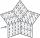# Permutations + divisibility - math problems

#### Number of problems found: 5

• Three numbersWe have three different non-zero digits. We will create all 3 digits numbers from them to use all 3 figures in each number. We add all the created numbers, and we get the sum of 1554. What were the numbers?
• Inverted nineIn the hotel Inverted Nine, each hotel room number is divisible by 6. How many rooms can we count with the three-digit number registered by digits 1,8,7,4,9?
• Digit sumHow many are three-digit numbers that have a digit sum of 6?
• PermutationsHow many 4-digit numbers can be composed of numbers 1,2,3,4,5,6,7 if: and the digits must not be repeated in the number b, the number should be divisible by five, and the numbers must not be repeated c, digits can be repeated
• RectanglesHow many rectangles with area 8713 cm2 whose sides are natural numbers are?

We apologize, but in this category are not a lot of examples.
Do you have an exciting math question or word problem that you can't solve? Ask a question or post a math problem, and we can try to solve it.

We will send a solution to your e-mail address. Solved examples are also published here. Please enter the e-mail correctly and check whether you don't have a full mailbox.

See also our permutations calculator. Permutations - math problems. Divisibility - math problems.